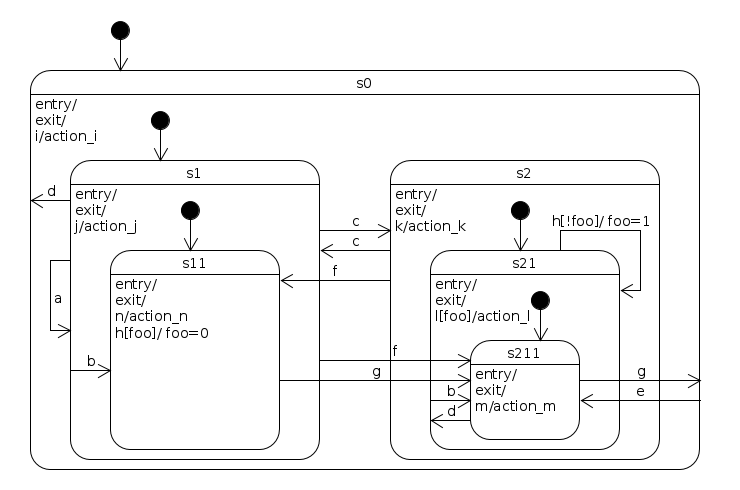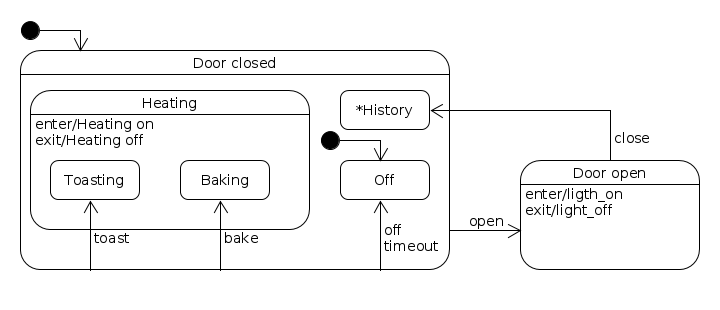# Examples¶

## Simple state machine¶

This is a simple state machine with only two states - on and off.

```from pysm import State, StateMachine, Event

on = State('on')
off = State('off')

sm = StateMachine('sm')

sm.initialize()

def test():
assert sm.state == on
sm.dispatch(Event('off'))
assert sm.state == off
sm.dispatch(Event('on'))
assert sm.state == on

if __name__ == '__main__':
test()
```

## Complex hierarchical state machine¶

A Hierarchical state machine similar to the one from Miro Samek’s book , page 95. It is a state machine that contains all possible state transition topologies up to four levels of state nesting```from pysm import State, StateMachine, Event

foo = True

def on_enter(state, event):
print('enter state {0}'.format(state.name))

def on_exit(state, event):
print('exit state {0}'.format(state.name))

def set_foo(state, event):
global foo
print('set foo')
foo = True

def unset_foo(state, event):
global foo
print('unset foo')
foo = False

def action_i(state, event):
print('action_i')

def action_j(state, event):
print('action_j')

def action_k(state, event):
print('action_k')

def action_l(state, event):
print('action_l')

def action_m(state, event):
print('action_m')

def action_n(state, event):
print('action_n')

def is_foo(state, event):
return foo is True

def is_not_foo(state, event):
return foo is False

m = StateMachine('m')
s0 = StateMachine('s0')
s1 = StateMachine('s1')
s2 = StateMachine('s2')
s11 = State('s11')
s21 = StateMachine('s21')
s211 = State('s211')

# Internal transitions

# External transition

# Attach enter/exit handlers
states = [m, s0, s1, s11, s2, s21, s211]
for state in states:
state.handlers = {'enter': on_enter, 'exit': on_exit}

m.initialize()

def test():
assert m.leaf_state == s11
m.dispatch(Event('a'))
assert m.leaf_state == s11
# This transition toggles state between s11 and s211
m.dispatch(Event('c'))
assert m.leaf_state == s211
m.dispatch(Event('b'))
assert m.leaf_state == s211
m.dispatch(Event('i'))
assert m.leaf_state == s211
m.dispatch(Event('c'))
assert m.leaf_state == s11
assert foo is True
m.dispatch(Event('h'))
assert foo is False
assert m.leaf_state == s11
# Do nothing if foo is False
m.dispatch(Event('h'))
assert m.leaf_state == s11
# This transition toggles state between s11 and s211
m.dispatch(Event('c'))
assert m.leaf_state == s211
assert foo is False
m.dispatch(Event('h'))
assert foo is True
assert m.leaf_state == s211
m.dispatch(Event('h'))
assert m.leaf_state == s211

if __name__ == '__main__':
test()
```

## Different ways to attach event handlers¶

A state machine and states may be created in many ways. The code below mixes many styles to demonstrate it (In production code you’d rather keep your code style consistent). One way is to subclass the `State` class and attach event handlers to it. This resembles the State Pattern way of writing a state machine. But handlers may live anywhere, really, and you can attach them however you want. You’re free to chose your own style of writing state machines with pysm. Also in this example a transition to a historical state is used.```import threading
import time
from pysm import StateMachine, State, Event

# It's possible to encapsulate all state related behaviour in a state class.
class HeatingState(StateMachine):
def on_enter(self, state, event):
oven = event.cargo['source_event'].cargo['oven']
if not oven.timer.is_alive():
oven.start_timer()
print('Heating on')

def on_exit(self, state, event):
print('Heating off')

def register_handlers(self):
self.handlers = {
'enter': self.on_enter,
'exit': self.on_exit,
}

class Oven(object):
TIMEOUT = 0.1

def __init__(self):
self.sm = self._get_state_machine()

def _get_state_machine(self):
oven = StateMachine('Oven')
door_closed = StateMachine('Door closed')
door_open = State('Door open')
heating = HeatingState('Heating')
toasting = State('Toasting')
baking = State('Baking')
off = State('Off')

# This time, a state behaviour is handled by Oven's methods.
door_open.handlers = {
'enter': self.on_open_enter,
'exit': self.on_open_exit,
'close': self.on_door_close
}

oven.initialize()
return oven

@property
def state(self):
return self.sm.leaf_state.name

def light_on(self):
print('Light on')

def light_off(self):
print('Light off')

def start_timer(self):
self.timer.start()

def bake(self):
self.sm.dispatch(Event('bake', oven=self))

def toast(self):
self.sm.dispatch(Event('toast', oven=self))

def open_door(self):
self.sm.dispatch(Event('open', oven=self))

def close_door(self):
self.sm.dispatch(Event('close', oven=self))

def on_timeout(self):
print('Timeout...')
self.sm.dispatch(Event('timeout', oven=self))

def on_open_enter(self, state, event):
print('Opening door')
self.light_on()

def on_open_exit(self, state, event):
print('Closing door')
self.light_off()

def on_door_close(self, state, event):
# Transition to a history state
self.sm.set_previous_leaf_state(event)

def test_oven():
oven = Oven()
print(oven.state)
assert oven.state == 'Off'
oven.bake()
print(oven.state)
assert oven.state == 'Baking'
oven.open_door()
print(oven.state)
assert oven.state == 'Door open'
oven.close_door()
print(oven.state)
assert oven.state == 'Baking'
time.sleep(0.2)
print(oven.state)
assert oven.state == 'Off'

if __name__ == '__main__':
test_oven()
```

## Reverse Polish notation calculator¶

A state machine is used in the Reverse Polish notation (RPN) calculator as a parser. A single event name (parse) is used along with specific inputs (See `pysm.pysm.StateMachine.add_transition()`).

This example also demonstrates how to use the stack of a state machine, so it behaves as a Pushdown Automaton (PDA)

```import string as py_string
from pysm import StateMachine, Event, State

class Calculator(object):
def __init__(self):
self.sm = self.get_state_machine()
self.result = None

def get_state_machine(self):
sm = StateMachine('sm')
initial = State('Initial')
number = State('BuildingNumber')
events=['parse'], input=py_string.digits,
action=self.start_building_number)
events=['parse'], input=py_string.digits,
action=self.build_number)
events=['parse'], input=py_string.whitespace)
events=['parse'], input='+-*/',
action=self.do_operation)
events=['parse'], input='=',
action=self.do_equal)
sm.initialize()
return sm

def parse(self, string):
for char in string:
self.sm.dispatch(Event('parse', input=char))

def calculate(self, string):
self.parse(string)
return self.result

def start_building_number(self, state, event):
digit = event.input
self.sm.stack.push(int(digit))
return True

def build_number(self, state, event):
digit = event.input
number = str(self.sm.stack.pop())
number += digit
self.sm.stack.push(int(number))
return True

def do_operation(self, state, event):
operation = event.input
y = self.sm.stack.pop()
x = self.sm.stack.pop()
# eval is evil
result = eval('float({0}) {1} float({2})'.format(x, operation, y))
self.sm.stack.push(result)
return True

def do_equal(self, state, event):
operation = event.input
number = self.sm.stack.pop()
self.result = number
return True

def test_calc_callbacks():
calc = Calculator()
assert calc.calculate(' 167 3 2 2 * * * 1 - =') == 2003
assert calc.calculate('    167 3 2 2 * * * 1 - 2 / =') == 1001.5
assert calc.calculate('    3   5 6 +  * =') == 33
assert calc.calculate('        3    4       +     =') == 7
assert calc.calculate('2 4 / 5 6 - * =') == -0.5

if __name__ == '__main__':
test_calc_callbacks()
```

Footnotes

  http://www.embedded.com/print/4008251 (visited on 07.06.2016)